# TruncatedDistribution distribution¶

class TruncatedDistribution(*args)

Truncated distribution.

Available constructors:

TruncatedDistribution(distribution, lowerBound, upperBound, threshold=0.5)

TruncatedDistribution(distribution, bound, side=ot.TruncatedDistribution.LOWER, threshold=0.5)

TruncatedDistribution(distribution, truncationInterval, threshold=0.5)

Parameters:
distributionDistribution

The distribution to be truncated.

lowerBound, upperBoundfloat

Define a new distribution range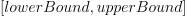with finite bounds. The upper bound must be strictly greater than the lower bound.

boundfloat

Scalar used when one of the bounds of the distribution is not finite.

If side = ot.TruncatedDistribution.LOWER: the interval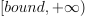is used to bound the distribution.

If side = ot.TruncatedDistribution.UPPER: the interval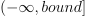is used to bound the distribution.

sideint, optional

Integer used when one of the bounds of the distribution is not finite. (Amongst ot.TruncatedDistribution.LOWER and ot.TruncatedDistribution.UPPER).

truncationIntervalInterval

Define a new distribution rangewith finite bounds.

thresholdfloat,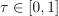This threshold is used when generating an evaluation of the distribution. We note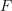the CDF of the non truncated distribution:

If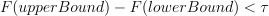: a CDF inversion is performed.

If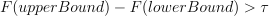: rejection.

Notes

Assuming a random variable X is truncated with two bounds a and b, its probability density function is defined as: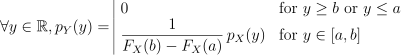and its cumulative density function is defined as:Examples

>>> import openturns as ot
>>> distribution = ot.TruncatedDistribution(ot.Normal(2.0, 1.5), 1.0, 4.0)


Draw a sample:

>>> sample = distribution.getSample(5)


Methods

 Transform distribution by absolute value function. Transform distribution by arccosine function. Transform distribution by acosh function. Transform distribution by arcsine function. Transform distribution by asinh function. Transform distribution by arctangent function. Transform distribution by atanh function. Transform distribution by cubic root function. Compute a bilateral confidence interval. Compute a bilateral confidence interval. computeCDF(*args) Compute the cumulative distribution function. computeCDFGradient(*args) Compute the gradient of the cumulative distribution function. Compute the characteristic function. Compute the complementary cumulative distribution function. Compute the conditional cumulative distribution function. Compute the conditional derivative density function of the last component. Compute the conditional probability density function. Compute the conditional quantile function of the last component. computeDDF(*args) Compute the derivative density function. computeDensityGenerator(betaSquare) Compute the probability density function of the characteristic generator. computeDensityGeneratorDerivative(betaSquare) Compute the first-order derivative of the probability density function. Compute the second-order derivative of the probability density function. Compute the entropy of the distribution. Compute the probability-generating function. Compute the inverse survival function. Compute the logarithm of the characteristic function. Compute the logarithm of the probability-generating function. computeLogPDF(*args) Compute the logarithm of the probability density function. Compute the gradient of the log probability density function. Compute the confidence interval with minimum volume. Compute the confidence interval with minimum volume. Compute the confidence domain with minimum volume. Compute the confidence domain with minimum volume. computePDF(*args) Compute the probability density function. computePDFGradient(*args) Compute the gradient of the probability density function. computeProbability(interval) Compute the interval probability. computeQuantile(*args) Compute the quantile function. computeRadialDistributionCDF(radius[, tail]) Compute the cumulative distribution function of the squared radius. computeScalarQuantile(prob[, tail]) Compute the quantile function for univariate distributions. Compute the sequential conditional cumulative distribution functions. Compute the sequential conditional derivative density function. Compute the sequential conditional probability density function. Compute the conditional quantile function of the last component. Compute the survival function. computeUnilateralConfidenceInterval(prob[, tail]) Compute a unilateral confidence interval. Compute a unilateral confidence interval. Transform distribution by cosine function. Transform distribution by cosh function. drawCDF(*args) Draw the cumulative distribution function. drawLogPDF(*args) Draw the graph or of iso-lines of log-probability density function. drawMarginal1DCDF(marginalIndex, xMin, xMax, ...) Draw the cumulative distribution function of a margin. drawMarginal1DLogPDF(marginalIndex, xMin, ...) Draw the log-probability density function of a margin. drawMarginal1DPDF(marginalIndex, xMin, xMax, ...) Draw the probability density function of a margin. drawMarginal1DSurvivalFunction(...[, logScale]) Draw the cumulative distribution function of a margin. drawMarginal2DCDF(firstMarginal, ...[, ...]) Draw the cumulative distribution function of a couple of margins. drawMarginal2DLogPDF(firstMarginal, ...[, ...]) Draw the log-probability density function of a couple of margins. drawMarginal2DPDF(firstMarginal, ...[, ...]) Draw the probability density function of a couple of margins. drawMarginal2DSurvivalFunction(...[, ...]) Draw the cumulative distribution function of a couple of margins. drawPDF(*args) Draw the graph or of iso-lines of probability density function. drawQuantile(*args) Draw the quantile function. Draw the cumulative distribution function. Transform distribution by exponential function. Set the bounds. Accessor to the CDF computation precision. Accessor to the componentwise central moments. Accessor to the Cholesky factor of the covariance matrix. Accessor to the object's name. Accessor to the copula of the distribution. (ditch me?) Accessor to the covariance matrix. Accessor to the componentwise description. Accessor to the dimension of the distribution. Dispersion indicator accessor. Get the distribution. Accessor to the object's id. Accessor to the number of Gauss integration points. Accessor to the inverse Cholesky factor of the covariance matrix. Accessor to the inverse iso-probabilistic transformation. Accessor to the iso-probabilistic transformation. Accessor to the Kendall coefficients matrix. Accessor to the componentwise kurtosis. getMarginal(*args) Accessor to marginal distributions. Accessor to the mean. Accessor to the componentwise moments. Accessor to the object's name. Accessor to the PDF computation precision. Accessor to the parameter of the distribution. Accessor to the parameter description of the distribution. Accessor to the number of parameters in the distribution. Accessor to the parameter of the distribution. Accessor to the Pearson correlation matrix. Position indicator accessor. Accessor to the discrete probability levels. Accessor to the range of the distribution. Accessor to a pseudo-random realization from the distribution. Accessor to roughness of the distribution. getSample(size) Accessor to a pseudo-random sample from the distribution. Accessor to a pseudo-random sample from the distribution. getSampleByQMC(size) Accessor to a low discrepancy sample from the distribution. Accessor to the object's shadowed id. Accessor to the shape matrix of the underlying copula if it is elliptical. getShiftedMoment(n, shift) Accessor to the componentwise shifted moments. Accessor to the singularities of the PDF function. Accessor to the componentwise skewness. Accessor to the Spearman correlation matrix. Accessor to the componentwise standard deviation. Accessor to the standard distribution. Accessor to the standard representative distribution in the parametric family. getSupport(*args) Accessor to the support of the distribution. Get the threshold used when generating an evaluation of the distribution. Accessor to the object's visibility state. Test whether the copula of the distribution is elliptical or not. Test whether the copula of the distribution is the independent one. Test if the object is named. Test if the object has a distinguishable name. Transform distribution by inverse function. Test whether the distribution is continuous or not. Test whether the distribution is a copula or not. Test whether the distribution is discrete or not. Test whether the distribution is elliptical or not. Test whether the distribution is integer-valued or not. Transform distribution by natural logarithm function. Transform distribution by natural logarithm function. setBounds(bounds) Set the bounds. setDescription(description) Accessor to the componentwise description. setDistribution(distribution) Set the distribution. setIntegrationNodesNumber(integrationNodesNumber) Accessor to the number of Gauss integration points. setName(name) Accessor to the object's name. setParameter(parameter) Accessor to the parameter of the distribution. Accessor to the parameter of the distribution. Accessor to the object's shadowed id. setThresholdRealization(thresholdRealization) Set the threshold used when generating an evaluation of the distribution. setVisibility(visible) Accessor to the object's visibility state. Transform distribution by sine function. Transform distribution by sinh function. Transform distribution by square function. Transform distribution by square root function. Transform distribution by tangent function. Transform distribution by tanh function.
 getCenteredMoment getSimplifiedVersion getStandardMoment
__init__(*args)
abs()

Transform distribution by absolute value function.

Returns:
distDistribution

The transformed distribution.

acos()

Transform distribution by arccosine function.

Returns:
distDistribution

The transformed distribution.

acosh()

Transform distribution by acosh function.

Returns:
distDistribution

The transformed distribution.

asin()

Transform distribution by arcsine function.

Returns:
distDistribution

The transformed distribution.

asinh()

Transform distribution by asinh function.

Returns:
distDistribution

The transformed distribution.

atan()

Transform distribution by arctangent function.

Returns:
distDistribution

The transformed distribution.

atanh()

Transform distribution by atanh function.

Returns:
distDistribution

The transformed distribution.

cbrt()

Transform distribution by cubic root function.

Returns:
distDistribution

The transformed distribution.

computeBilateralConfidenceInterval(prob)

Compute a bilateral confidence interval.

Parameters:
alphafloat,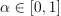The confidence level.

Returns:
confIntervalInterval

The confidence interval of level.

Notes

We consider an absolutely continuous measure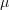with density function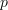.

The bilateral confidence interval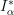is the cartesian product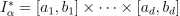where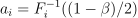and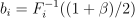for alland which verifies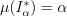.

Examples

Create a sample from a Normal distribution:

>>> import openturns as ot
>>> sample = ot.Normal().getSample(10)
>>> ot.ResourceMap.SetAsUnsignedInteger('DistributionFactory-DefaultBootstrapSize', 100)


Fit a Normal distribution and extract the asymptotic parameters distribution:

>>> fittedRes = ot.NormalFactory().buildEstimator(sample)
>>> paramDist = fittedRes.getParameterDistribution()


Determine the bilateral confidence interval at level 0.9:

>>> confInt = paramDist.computeBilateralConfidenceInterval(0.9)

computeBilateralConfidenceIntervalWithMarginalProbability(prob)

Compute a bilateral confidence interval.

Parameters:
alphafloat,The confidence level.

Returns:
confIntervalInterval

The confidence interval of level.

marginalProbfloat

The value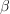which is the common marginal probability of each marginal interval.

Examples

Create a sample from a Normal distribution:

>>> import openturns as ot
>>> sample = ot.Normal().getSample(10)
>>> ot.ResourceMap.SetAsUnsignedInteger('DistributionFactory-DefaultBootstrapSize', 100)


Fit a Normal distribution and extract the asymptotic parameters distribution:

>>> fittedRes = ot.NormalFactory().buildEstimator(sample)
>>> paramDist = fittedRes.getParameterDistribution()


Determine the bilateral confidence interval at level 0.9 with marginal probability:

>>> confInt, marginalProb = paramDist.computeBilateralConfidenceIntervalWithMarginalProbability(0.9)

computeCDF(*args)

Compute the cumulative distribution function.

Parameters:
Xsequence of float, 2-d sequence of float

CDF input(s).

Returns:
Ffloat, Point

CDF value(s) at input(s)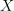.

Notes

The cumulative distribution function is defined as: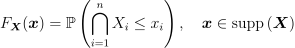Compute the gradient of the cumulative distribution function.

Parameters:
Xsequence of float

CDF input.

Returns:
dFdthetaPoint

Partial derivatives of the CDF with respect to the distribution parameters at input.

computeCharacteristicFunction(*args)

Compute the characteristic function.

Parameters:
tfloat

Characteristic function input.

Returns:
phicomplex

Characteristic function value at input.

Notes

The characteristic function is defined as: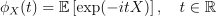OpenTURNS features a generic implementation of the characteristic function for all its univariate distributions (both continuous and discrete). This default implementation might be time consuming, especially as the modulus ofgets high. Only some univariate distributions benefit from dedicated more efficient implementations.

computeComplementaryCDF(*args)

Compute the complementary cumulative distribution function.

Parameters:
Xsequence of float, 2-d sequence of float

Complementary CDF input(s).

Returns:
Cfloat, Point

Complementary CDF value(s) at input(s).

Notes

The complementary cumulative distribution function.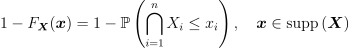Warning

This is not the survival function (except for 1-dimensional distributions).

computeConditionalCDF(*args)

Compute the conditional cumulative distribution function.

Parameters:
Xnfloat, sequence of float

Conditional CDF input (last component).

Xcondsequence of float, 2-d sequence of float with size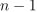Conditionning values for the other components.

Returns:
Ffloat, sequence of float

Conditional CDF value(s) at input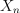,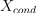.

Notes

The conditional cumulative distribution function of the last component with respect to the other fixed components is defined as follows: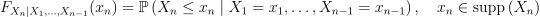computeConditionalDDF(x, y)

Compute the conditional derivative density function of the last component.

With respect to the other fixed components.

Parameters:
Xnfloat

Conditional DDF input (last component).

Xcondsequence of float with dimensionConditionning values for the other components.

Returns:
dfloat

Conditional DDF value at input,.

computeConditionalPDF(*args)

Compute the conditional probability density function.

Conditional PDF of the last component with respect to the other fixed components.

Parameters:
Xnfloat, sequence of float

Conditional PDF input (last component).

Xcondsequence of float, 2-d sequence of float with sizeConditionning values for the other components.

Returns:
Ffloat, sequence of float

Conditional PDF value(s) at input,.

computeConditionalQuantile(*args)

Compute the conditional quantile function of the last component.

Conditional quantile with respect to the other fixed components.

Parameters:
pfloat, sequence of float,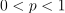Conditional quantile function input.

Xcondsequence of float, 2-d sequence of float with sizeConditionning values for the other components.

Returns:
X1float

Conditional quantile at input,.

computeDDF(*args)

Compute the derivative density function.

Parameters:
Xsequence of float, 2-d sequence of float

PDF input(s).

Returns:
d

DDF value(s) at input(s).

Notes

The derivative density function is the gradient of the probability density function with respect to: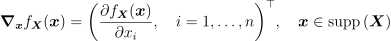computeDensityGenerator(betaSquare)

Compute the probability density function of the characteristic generator.

PDF of the characteristic generator of the elliptical distribution.

Parameters:
beta2float

Density generator input.

Returns:
pfloat

Density generator value at input.

Notes

This is the function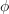such that the probability density function rewrites: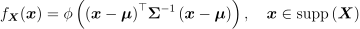This function only exists for elliptical distributions.

computeDensityGeneratorDerivative(betaSquare)

Compute the first-order derivative of the probability density function.

PDF of the characteristic generator of the elliptical distribution.

Parameters:
beta2float

Density generator input.

Returns:
pfloat

Density generator first-order derivative value at input.

Notes

This function only exists for elliptical distributions.

computeDensityGeneratorSecondDerivative(betaSquare)

Compute the second-order derivative of the probability density function.

PDF of the characteristic generator of the elliptical distribution.

Parameters:
beta2float

Density generator input.

Returns:
pfloat

Density generator second-order derivative value at input.

Notes

This function only exists for elliptical distributions.

computeEntropy()

Compute the entropy of the distribution.

Returns:
efloat

Entropy of the distribution.

Notes

The entropy of a distribution is defined by: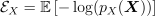Where the random vector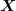follows the probability distribution of interest, and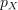is either the probability density function ofif it is continuous or the probability distribution function if it is discrete.

computeGeneratingFunction(*args)

Compute the probability-generating function.

Parameters:
zfloat or complex

Probability-generating function input.

Returns:
gfloat

Probability-generating function value at input.

Notes

The probability-generating function is defined as follows: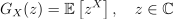This function only exists for discrete distributions. OpenTURNS implements this method for univariate distributions only.

computeInverseSurvivalFunction(point)

Compute the inverse survival function.

Parameters:
pfloat,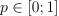Level of the survival function.

Returns:
xPoint

Pointsuch that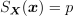with iso-quantile components.

Notes

The inverse survival function writes: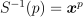where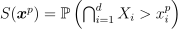. OpenTURNS returns the point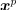such that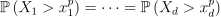.

computeLogCharacteristicFunction(*args)

Compute the logarithm of the characteristic function.

Parameters:
tfloat

Characteristic function input.

Returns:
phicomplex

Logarithm of the characteristic function value at input.

Notes

OpenTURNS features a generic implementation of the characteristic function for all its univariate distributions (both continuous and discrete). This default implementation might be time consuming, especially as the modulus ofgets high. Only some univariate distributions benefit from dedicated more efficient implementations.

computeLogGeneratingFunction(*args)

Compute the logarithm of the probability-generating function.

Parameters:
zfloat or complex

Probability-generating function input.

Returns:
lgfloat

Logarithm of the probability-generating function value at input.

Notes

This function only exists for discrete distributions. OpenTURNS implements this method for univariate distributions only.

computeLogPDF(*args)

Compute the logarithm of the probability density function.

Parameters:
Xsequence of float, 2-d sequence of float

PDF input(s).

Returns:
ffloat, Point

Logarithm of the PDF value(s) at input(s).

Compute the gradient of the log probability density function.

Parameters:
Xsequence of float

PDF input.

Returns:
dfdthetaPoint

Partial derivatives of the logPDF with respect to the distribution parameters at input.

computeMinimumVolumeInterval(prob)

Compute the confidence interval with minimum volume.

Parameters:
alphafloat,The confidence level.

Returns:
confIntervalInterval

The confidence interval of level.

Notes

We consider an absolutely continuous measurewith density function.

The minimum volume confidence intervalis the cartesian productwhere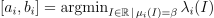andwith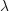is the Lebesgue measure on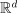.

This problem resorts to solving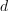univariate non linear equations: for a fixed value, we find each intervals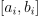such that: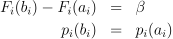which consists of finding the bound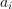such that: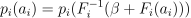To find, we use the Brent algorithm: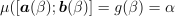with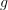a non linear function.

Examples

Create a sample from a Normal distribution:

>>> import openturns as ot
>>> sample = ot.Normal().getSample(10)
>>> ot.ResourceMap.SetAsUnsignedInteger('DistributionFactory-DefaultBootstrapSize', 100)


Fit a Normal distribution and extract the asymptotic parameters distribution:

>>> fittedRes = ot.NormalFactory().buildEstimator(sample)
>>> paramDist = fittedRes.getParameterDistribution()


Determine the confidence interval of the native parameters at level 0.9 with minimum volume:

>>> ot.ResourceMap.SetAsUnsignedInteger('Distribution-MinimumVolumeLevelSetSamplingSize', 1000)
>>> confInt = paramDist.computeMinimumVolumeInterval(0.9)

computeMinimumVolumeIntervalWithMarginalProbability(prob)

Compute the confidence interval with minimum volume.

Parameters:
alphafloat,The confidence level.

Returns:
confIntervalInterval

The confidence interval of level.

marginalProbfloat

The valuewhich is the common marginal probability of each marginal interval.

Examples

Create a sample from a Normal distribution:

>>> import openturns as ot
>>> sample = ot.Normal().getSample(10)
>>> ot.ResourceMap.SetAsUnsignedInteger('DistributionFactory-DefaultBootstrapSize', 100)


Fit a Normal distribution and extract the asymptotic parameters distribution:

>>> fittedRes = ot.NormalFactory().buildEstimator(sample)
>>> paramDist = fittedRes.getParameterDistribution()


Determine the confidence interval of the native parameters at level 0.9 with minimum volume:

>>> ot.ResourceMap.SetAsUnsignedInteger('Distribution-MinimumVolumeLevelSetSamplingSize', 1000)
>>> confInt, marginalProb = paramDist.computeMinimumVolumeIntervalWithMarginalProbability(0.9)

computeMinimumVolumeLevelSet(prob)

Compute the confidence domain with minimum volume.

Parameters:
alphafloat,The confidence level.

Returns:
levelSetLevelSet

The minimum volume domain of measure.

Notes

We consider an absolutely continuous measurewith density function.

The minimum volume confidence domain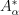is the set of minimum volume and which measure is at least. It is defined by: